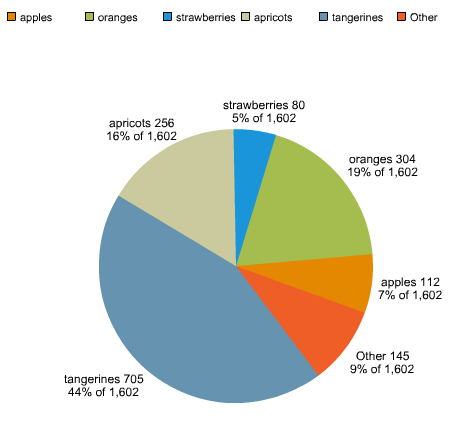Two days ago I wrote about a pie chart that had too much data. As you can see in the blog post, the amount of data and size of the pie chart causes the labels to run in together. It's also just a bit too much for a pie. As one commenter said, a bar char would be better, or as another said, it may make sense to combine some of the smaller slices into an "Other" slice. In this post I do exactly that. Given a random query I'm going to see if I have too many data points and if so - I'll combine them into a new slice called Other. I'm going to begin with the assumption that q, our query, already exists. At the very end of the blog post I'll put up the entire code template in case you want to try this yourself.

First - I chose 6 as the max number of slices I wanted. You can go higher or lower of course.

`<cfif q.recordCount gt 6> <!--- number of items we want to strip/join ---> <cfset otherCount = q.recordCount - 5>`

This code block shows my initial check to see if I have too much data. If I do, I create a variable called otherCount. This is how many rows of data that will be cominbed into the other slice. Notice it is 5, not 6. Remember that I'm adding a new slice, Other. So I want to leave 6-1 slices of data. Now let's get the data that will fall into the Other block.

`<cfquery name="getOthers" dbtype="query" maxrows="#otherCount#"> select fruit, total from q order by total asc </cfquery>`

Nothing crazy here - just a query of query. I sort by total asc as I want to combine the smallest items. Now let's get their total:

`<!--- total of Other ---> <cfset otherTotal = arraySum(listToArray(valueList(getOthers.total)))>`

And then let's get the query minus the rows we took out.

`<!--- query without others ---> <cfquery name="newData" dbtype="query"> select * from q where fruit not in (<cfqueryparam cfsqltype="cf_sql_varchar" value="#valueList(getOthers.fruit)#" list="true">) </cfquery>`

Now we need to add in our new slice:

`<!--- add Other ---> <cfset queryAddRow(newData)> <cfset querySetCell(newData, "fruit", "Other")> <cfset querySetCell(newData, "total", otherTotal)>`

And finally - copy over newData:

`<cfset q = newData> </cfif>`

And here is the final result - compare it to the screen shots in the previous post:Neat! So here is the complete template. Enjoy.

`<cfset q = queryNew("fruit,total","cf_sql_varchar,cf_sql_integer")> <cfset queryAddRow(q)> <cfset querySetCell(q, "fruit","apples")> <cfset querySetCell(q, "total",112)> <cfset queryAddRow(q)> <cfset querySetCell(q, "fruit","oranges")> <cfset querySetCell(q, "total",304)> <cfset queryAddRow(q)> <cfset querySetCell(q, "fruit","bananas")> <cfset querySetCell(q, "total",0)> <cfset queryAddRow(q)> <cfset querySetCell(q, "fruit","pears")> <cfset querySetCell(q, "total",0)> <cfset queryAddRow(q)> <cfset querySetCell(q, "fruit","grapes")> <cfset querySetCell(q, "total",16)> <cfset queryAddRow(q)> <cfset querySetCell(q, "fruit","strawberries")> <cfset querySetCell(q, "total",80)> <cfset queryAddRow(q)> <cfset querySetCell(q, "fruit","plums")> <cfset querySetCell(q, "total",48)> <cfset queryAddRow(q)> <cfset querySetCell(q, "fruit","pineapples")> <cfset querySetCell(q, "total",32)> <cfset queryAddRow(q)> <cfset querySetCell(q, "fruit","blueberries")> <cfset querySetCell(q, "total",16)> <cfset queryAddRow(q)> <cfset querySetCell(q, "fruit","raspberries")> <cfset querySetCell(q, "total",32)> <cfset queryAddRow(q)> <cfset querySetCell(q, "fruit","apricots")> <cfset querySetCell(q, "total",256)> <cfset queryAddRow(q)> <cfset querySetCell(q, "fruit","tangerines")> <cfset querySetCell(q, "total",705)> <cfset queryAddRow(q)> <cfset querySetCell(q, "fruit","cherries")> <cfset querySetCell(q, "total",1)> <cfset queryAddRow(q)> <cfset querySetCell(q, "fruit","peaches")> <cfset querySetCell(q, "total",0)>`

`<cfif q.recordCount gt 6> <!--- number of items we want to strip/join ---> <cfset otherCount = q.recordCount - 5> <cfquery name="getOthers" dbtype="query" maxrows="#otherCount#"> select fruit, total from q order by total asc </cfquery> <!--- total of Other ---> <cfset otherTotal = arraySum(listToArray(valueList(getOthers.total)))>`

`<!--- query without others ---> <cfquery name="newData" dbtype="query"> select * from q where fruit not in (<cfqueryparam cfsqltype="cf_sql_varchar" value="#valueList(getOthers.fruit)#" list="true">) </cfquery>`

`<!--- add Other ---> <cfset queryAddRow(newData)> <cfset querySetCell(newData, "fruit", "Other")> <cfset querySetCell(newData, "total", otherTotal)>`

`<cfset q = newData> </cfif>`

`<cfchart chartheight="500" chartwidth="500"> <cfchartseries type="pie" query="q" itemcolumn="fruit" valuecolumn="total" datalabelstyle="pattern" > </cfchart>`